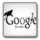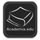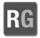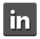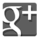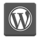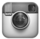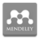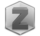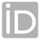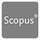You are in:Home/Publications/On The Numerical Solution of Partial integro-differential equations

### Dr. amal foad abdelaziz soliman :: Publications:

 Title: On The Numerical Solution of Partial integro-differential equations Authors: A. F. Soliman; A M.A. EL-ASYED; M. S. El-Azab Year: 2012 Keywords: Compact finite difference method; PIDE; Time discretization; Partial integro-differential equations; High accuracy; Collocation method. Journal: Mathematical Sciences Letters Volume: 1 Issue: Not Available Pages: 71-80 Publisher: Not Available Local/International: International Paper Link: Not Available Full paper amal foad abdelaziz soliman_p4.pdf Supplementary materials Not Available
 Abstract: In this paper, we consider the approximate solution of the partial integro-differential equation. To solve this problem, we introduce a new nonstandard time discretization scheme. Then the fourth order finite difference and collocation method is presented for the numerical solution of this type of partial integro-differential equation (PIDE). A composite weighted trapezoidal rule is manipulated to handle the numerical integrations which results in a closed-form difference scheme. The efficiency and accuracy of the scheme is validated by its application to one test problem which have exact solutions. Numerical results show that this fourth-order scheme has the expected accuracy. The most advantages of compact finite difference method for PIDE are that it obtains high order of accuracy, while the time complexity to solve the matrix equations after we use compact finite difference method on PIDE is O(N), and it can solve very general case of PIDE.